## सी. बी. एस. ई. गणित में सबसे महत्वपूर्ण प्रश्न पत्र - 2018 नमूना प्रश्न

The question paper consist of 26 questions divided into three sections A, B and C. Section A comprises of 6 questions of one mark each, section B comprises of 13 questions of four marks each and section C comprises of 07 questions of six marks each.

#### SECTION A (1 Mark )

`Q.1` Prove that:`Q.2``Q.3` Find the value of p if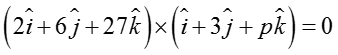`Q.4`if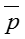is a unit vectors and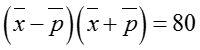`Q.5` Find the value of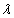so that the two vectors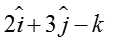andare parallel.

`Q.6`6. Let * be a binary operation on the set I of integers, defined by a * b = 2a + b - 3. Find the value of 3 * 4.

#### SECTION B (2 Marks )

`Q.7` Let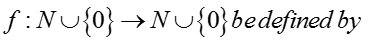. Show that f is bijection.

`Q.8` Prove thatOR

Solve`Q.9` 9. A window in the form of a rectangle is surmounted by a semi-circular opening. The total perimeter of the window is 10 m. Find the dimensions of the rectangular part of the window to admit maximum light through the whole opening.

`Q.10` If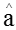and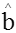are unit vectors inclined at an angle θ then prove that`Q.11` If a,b and c are in A.P. Prove that.

`Q.12`.

OR

Solve the equations :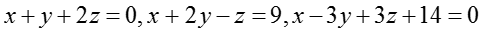`Q.13`OR`Q.14``Q.15``Q.16``Q.17` Ifthan proveOR

If y =, than show that`Q.18` A, B, C in order draws a card from a pack of cards, replacing them after each draw, on condition that the first who draws a spade shall win a prize : find their respective chances.

`Q.19` If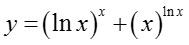, than find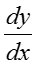#### SECTION C (3 Marks )

`Q.20` Find the image of the point (3, 5, 3) in the line.

OR

Find the distance of the point (-2, 3, -4) from the linemeasured parallel to the plane 4x + 12y – 3z + 1 = 0.

`Q.21`Using integration , find the area bounded by the lines x + 2y = 2, y – x = 1 and 2x + y = 7.

`Q.22` Solve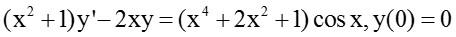.

OR

Solve.

`Q.23` 23. In a hurdle race, a player has to cross 10 hurdles. The probability that he will clear each hurdle is 5/6.What is the probability that he will knock down fewer than 2 hurdles ?

`Q.24` 24. Vikas has been given two lists of problems from his mathematics teacher with the instructions to submit not more than 100 of them correctly solved for marks. The problems in the first list are worth 10 marks each and those in the second list are worth 5 marks each. Vikas knows from past experience that he requires on an average of 4 minutes to solve a problem of 10 marks and 2 minutes to solve a problem of 5 marks. He has other subjects to worry about; he cannot devote more than 4 hours to his mathematics assignment. With reference to manage his time in best possible way how many problems from each list shall he do to maximize his marks? What is the importance of time management for students?

`Q.25` A window is in the form of a rectangle surmounted by a semi-circular opening. The total perimeter of the window is 10 m, find the dimensions of the window to admit maximum light through the whole opening

`Q.26` Evaluate the following integrals as limit of sums: